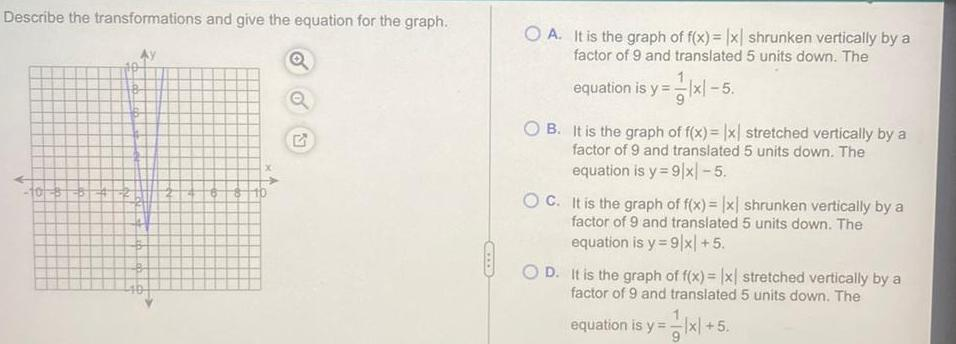Question:

# Describe the transformations and give the equation for the

Last updated: 11/11/2023Describe the transformations and give the equation for the graph 10 PPA K 111 8 6 8 A 2 AIXS OA It is the graph of f x x shrunken vertically by a factor of 9 and translated 5 units down The equation is y xl 5 OB It is the graph of f x x stretched vertically by a factor of 9 and translated 5 units down The equation is y 9 x 5 OC It is the graph of f x x shrunken vertically by a factor of 9 and translated 5 units down The equation is y 9 x 5 OD It is the graph of f x x stretched vertically by a factor of 9 and translated 5 units down The equation is y x 5